# FALSE In ExcelArticle byExcelMojo Team## What Is FALSE In Excel?

The FALSE Excel Function returns the Boolean logical value FALSE. We can with or without the parenthesis. The function is useful with IF() and IFERROR() functions than on its own. The Excel FALSE function is an inbuilt Logical function, so we can insert the formula from the “Function Library” or enter it directly in the worksheet.

For example, the table below shows scores out of 100, and we will determine the status of the full marks using the FALSE in Excel and the IF Excel function.

Select cell B2, enter the formula =IF(A2=100,”Full Marks”,FALSE()), press “Enter”, and drag the formula from cell B2 to B11 using the Excel fill handle.

We get the output shown above. Here, if the given score equals 100, the IF() function returns the output as “Full Marks”, otherwise, updates the status as FALSE. Column C is for our reference.

###### Key Takeaways
• The function FALSE in Excel output is the logical value FALSE. Users can apply the FALSE function in a cell or formula to display the value FALSE.
• The FALSE function does not take any arguments as input. So, we can enter the formula with or without the brackets.
• We can use the FALSE() as a standalone function. However, since it is a compatibility function, it is best to use it with other Excel logical functions, such as IF, IFERROR, SUMIF, and COUNTIF.

### FALSE() Excel Formula

The syntax of the FALSE in Excel is,

The FALSE() does not take any arguments as input.

### How To Use FALSE Excel Function?

We can use the FALSE Excel Function in 2 ways, namely,

• Access from the Excel ribbon.
• Enter in the worksheet manually.

#### Method #1 – Access from the Excel ribbon

First, choose an empty cell for output → select the “Formulas” tab → go to the “Function Library” group → click the “Logical” option drop-down → select the “FALSE” function, as shown below.

The “Function Arguments” window appears. Since the function doesn’t accept any argument, just click “OK”, as shown below.

#### Method #2 – Enter in the worksheet manually

1. First, select an empty cell for the output.
2. Next, type =FALSE( in the selected cell. [Alternatively, type =F and double-click on the FALSE function from the Excel suggestions.]
3. Finally, press Enter to execute the formula.

Let us take a basic example to understand this function.

We will use the FALSE in Excel and find whether the given conditions are True or False.

The table below contains condition statements.

The steps to find the logical value using the FALSE in Excel are,

1. We know the given condition statement in cell A2 is FALSE. So select cell B2, enter the formula =FALSE() as a standalone function, and press “Enter”. The result is FALSE, as shown below.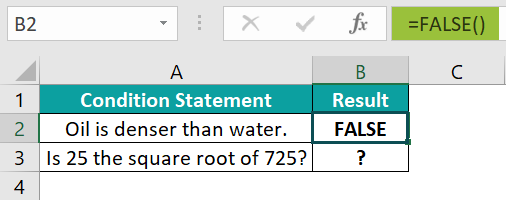[Alternatively, we can enter the FALSE() using the option available in the Formulas tab. And for that, we must select the target cell and click FormulasLogicalFALSE.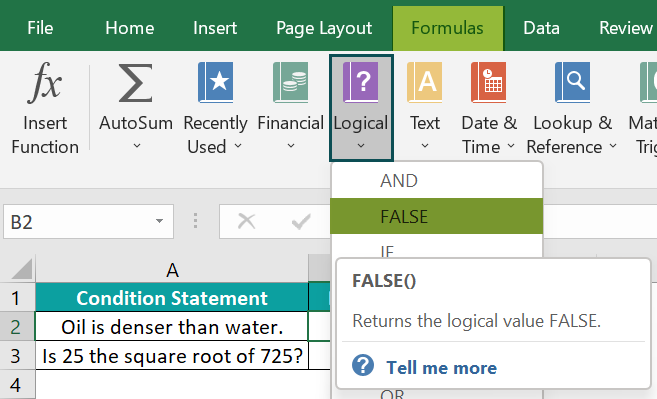The above action will open the Function Arguments window. But, as we do not need to enter any argument values, the window will provide the FALSE in Excel and show the expected result.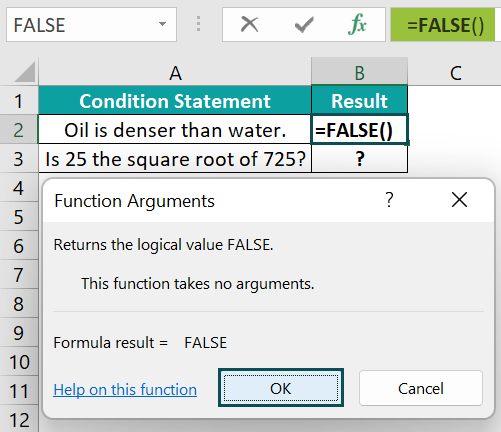And once we click OK, the FALSE() gets executed in the target cell.]

2. As the second condition statement is a question, we will use the FALSE() within the IF() function.

So, select cell B3, enter the formula =IF(SQRT(725)=25,”TRUE”,FALSE()), and press Enter.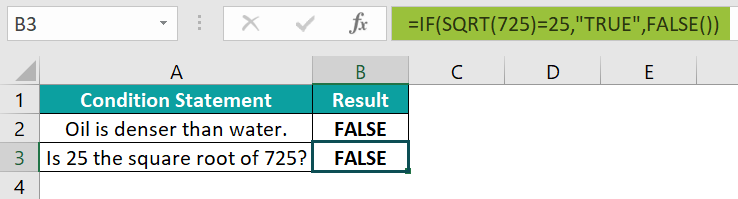We get the above output.

[Output Observation: In the above formula, the SQRT() returns the square root of the value 725, 26.925. The IF() then checks if the value 26.925 equals 25. And as the condition does not hold, the false_value or the FALSE() gets executed. And hence the IF() returns FALSE as the output in the target cell.]

### Examples

We will understand advanced scenarios using the FALSE in Excel examples.

#### Example #1

We will use the FALSE() with IF Excel Function for the data involving string values.

The table below shows the order and shipment data of a list of laptops, and we will determine the demand fulfillment status.

The steps to apply the FALSE in Excel within the IF() are,

• Step 1: Select cell E2, enter the formula =IF(AND(B2<C2,D2=”Yes”),TRUE,FALSE()), and press Enter. We will get the result as TRUE, as shown below.

[Note: The IF() checks two conditions. The first condition is that the units ordered should be more than the units required, and the second one is that the order shipped status should be Yes.]

• Step 2: Drag the formula from cell E2 to E11 using the fill handle.

We get the output shown above.

[Output Observation: Let us consider the cell E11 formula to see how the formula works. As the first condition does not hold, the function FALSE in Excel gets executed, and the IF() returns the demand met status as FALSE.]

#### Example #2

We will use the FALSE() with COUNTIF Function in excel for the data where not all state-capital pairs are correct, and we will count the number of mismatches.

The following table contains a list of US states and capitals in columns A and B, and column C shows the match.

The procedure to apply the FALSE in Excel within the COUNTIF() is,

Select cell B13, enter the formula =COUNTIF(C2:C10,FALSE()), and press Enter.

The output is shown above.

[Output Observation: The given criteria is to match the FALSE() return value. So, the COUNTIF() counts the number of FALSE values in the specified range, C2:C10, and returns the count as 6.]

#### Example #3

We will use the FALSE() within the SUMIF function in Excel and calculate the total quantity of fruits that are not Category A.

The below table contains a list of fruits, their category, and quantity details.

The procedure to apply the SUMIF() with the function FALSE in Excel is,

Select cell B13, enter the formula =SUMIF(B2:B11,FALSE(),C2:C11), and press Enter.

We get the above output.

[Output Observation: First, the given criterion is FALSE(). So, the criterion in the above formula is the logical value FALSE. Next, the SUMIF() checks for the FALSE values in the range B2:B11. The cells B3, B5, B6, B7, and B11 satisfy the condition. And thus, the function adds the quantity values in the corresponding column C cells to return the required total quantity as 9800.]

### Important Things To Note

• The function FALSE in Excel and the logical value FALSE is the same.
• The numeric value of the FALSE() output is 0.
• Though we can directly enter FALSE in a cell or formula, the FALSE function affirms compatibility with other spreadsheet software packages.

1. Where is the FALSE function in Excel?

First, choose an empty cell for output → select the “Formulas” tab → go to the “Function Library” group → click the “Logical” option drop-down → select the “FALSE” function, as shown below.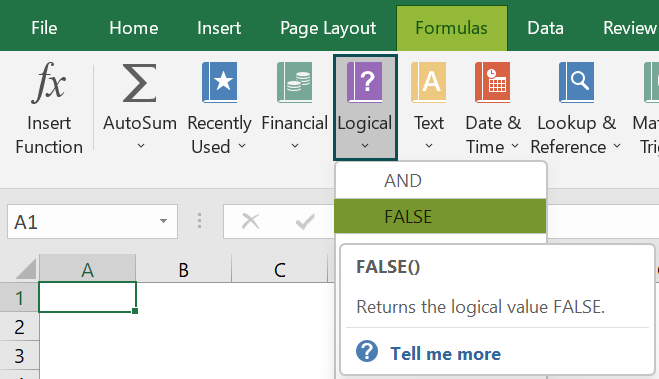2. How to use the FALSE function with IFERROR()?

We can use the FALSE function with IFERROR() to determine the price per unit in column C and display the result as FALSE in cells where the division of the two given values results in an error.

For example, the following table shows inventory data.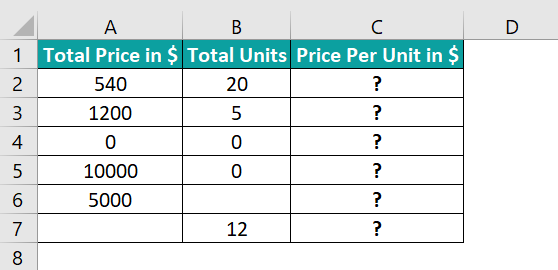The steps to apply the IFERROR() with FALSE() are,

• Step 1: Select cell C2, enter the formula =IFERROR(A2/B2,FALSE()), and press Enter. The result is 27, as shown below.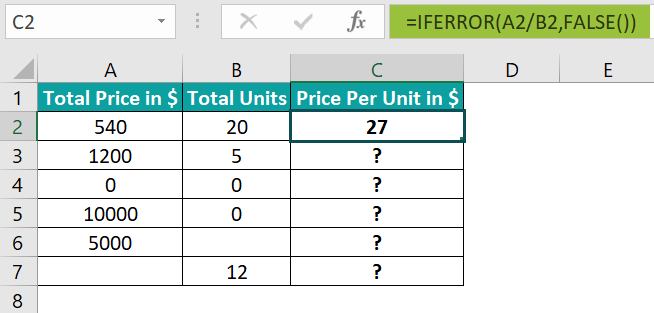• Step 2: Drag the formula from cell C2 to C7 using the fill handle.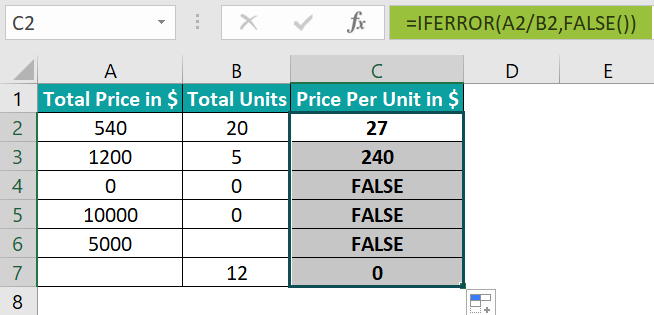We get the output shown above.

[Output Observation: As the total units are 0 in rows 4 and 5 and missing in row 6 data, the IFERROR() value arguments in the respective target cells result in the #DIV/0! error. And hence, the IFERROR() returns the value_if_error argument value as the output. So, the FALSE() gets executed, and thus, we get the logical value FALSE as the output in the target cells C4:C6.]

3. Can we perform arithmetic operations using the FALSE function in Excel?

We can perform arithmetic operations using the FALSE in Excel, as the FALSE() numeric value is 0.

For example, the below table contains a range of values in the Value 1 column, and Value 2 is the FALSE(). Column C shows the arithmetic operation to perform in the corresponding column D cells using the values 1 and 2.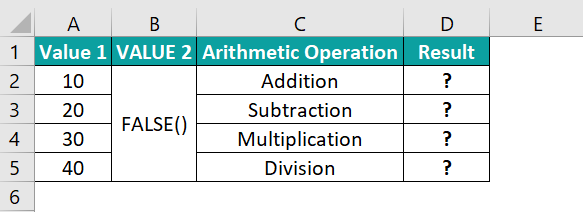The steps to apply the required formulas using the FALSE() in column D are,

• Step 1: Select cell D2, enter the formula =A2+FALSE(), and press Enter. The result is 10.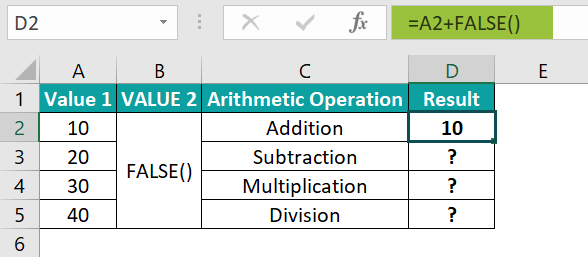• Step 2: Select cell D3, enter the formula =A3-FALSE(), and press Enter. The result is 20.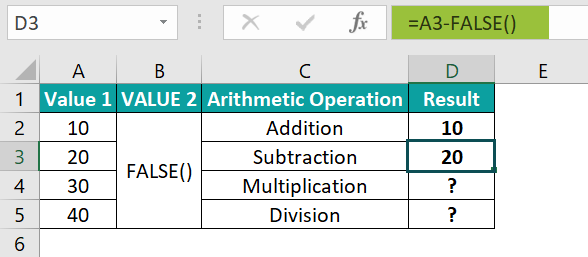• Step 3: Select cell D4, enter the formula =A4*FALSE(), and press Enter. The result is 0.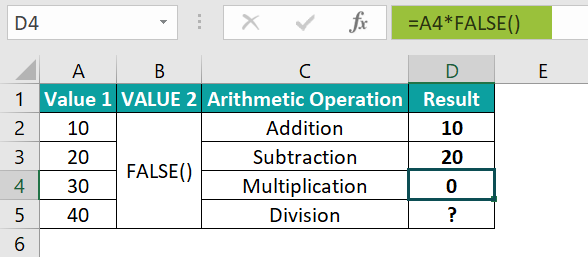• Step 4: Select cell D5, enter the formula =A5/FALSE(), and press Enter. The result is #DIV/0!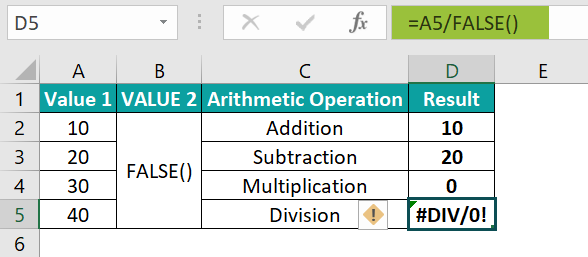Output Observation: The formula in each target cell takes the numeric value of the FALSE function as 0. And hence, in cell D2, the formula performs the operation 10+0, resulting in the value 10. Likewise, in cells D3 and D4, the arithmetic operations performed are 20-0 and 30*0, resulting in 20 and 0 in the respective target cells.

Finally, the expression executed in cell D5 is 40/0. And as the denominator is 0, the division operation results in the #DIV/0! error.